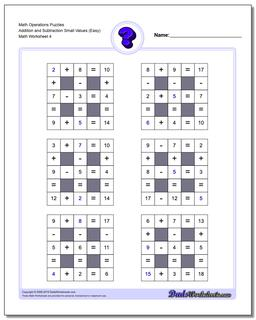One Dad. Four daughters. 9,341 worksheets... and counting!# Math Operations Puzzles Addition and Subtraction Small Values (Easy) (Fourth Worksheet)

PropertyValue
DescriptionMath Operations Puzzles Addition and Subtraction Small Values (Easy): Math puzzle worksheets that require students to fill in missing values (Smaller Numbers). (Fourth Worksheet)
Resource TypeWorksheet